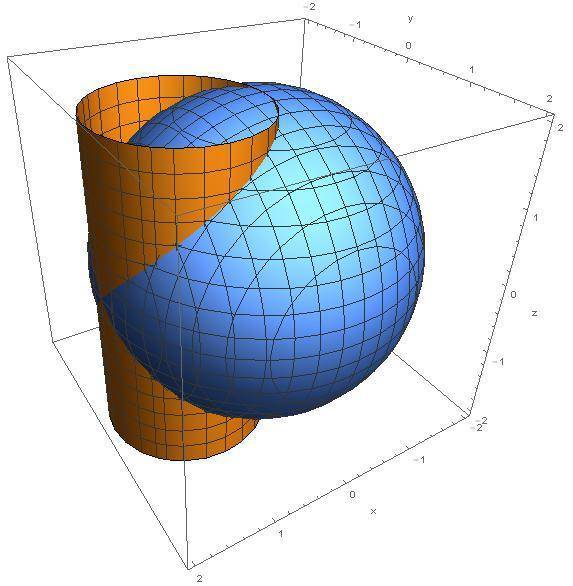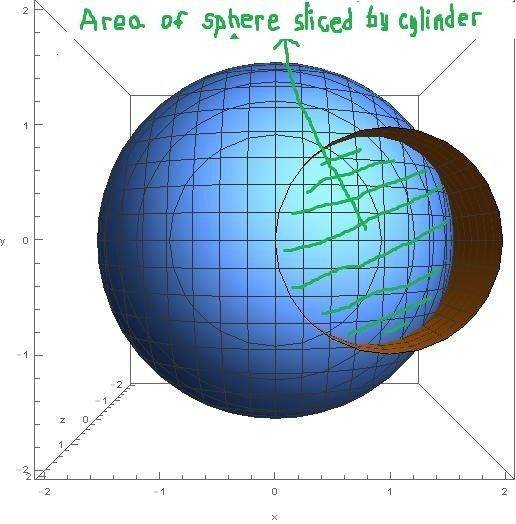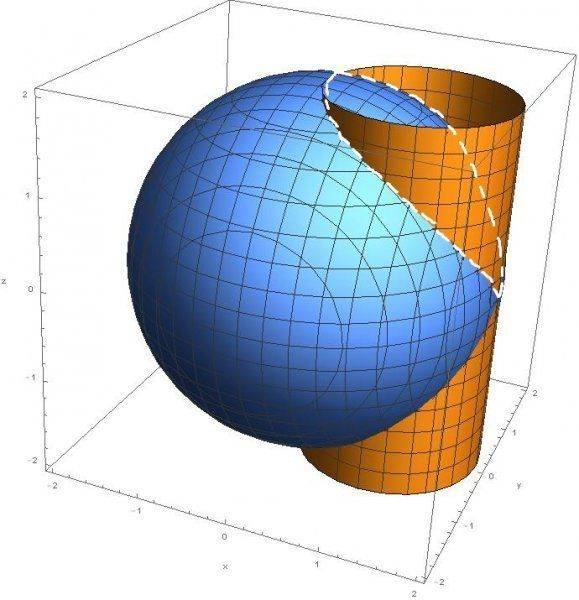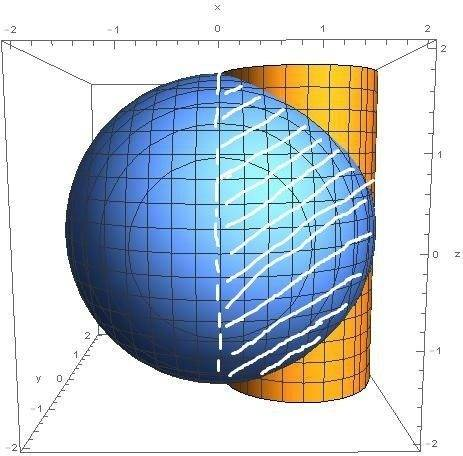# Area of cylinder sliced by sphere

Hi!
Calculate area of cylinder $$x^{2}+y^{x}=ax$$ sliced by sphere $$x^{2}+y^{2}+z^{2}=a^{2}$$.
Here is graph:How to do it? If problem was "Calculate area of sphere $$x^{2}+y^{2}+z^{2}=a^{2}$$ sliced by cylinder $$x^{2}+y^{x}=ax$$" I would solve it using double integrals ($$P=\iint\sqrt{1+(\frac{\partial z}{\partial x})^{2}+(\frac{\partial z}{\partial y})^{2}}dxdy$$ over region $$x^{2}+y^{x}=ax$$). That area would be this (I think):But what would be area of cylinder sliced by sphere? Maybe this:Last edited: